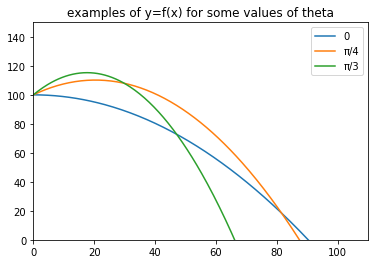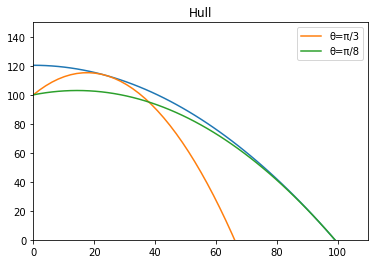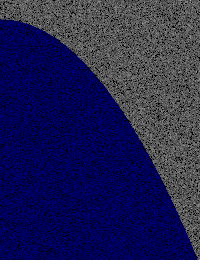# Ballistics and calculus

I'm a bit of a fan of math puzzles. There is a well-known website publishing puzzles and one of them brought me some fun for a while.

I'm not naming the website on purpose, but you can easily find it. It's named after a famous mathematician who ended up almost blind. Fortunately, he had memorized most of his books before.

You won't find a numeric solution here, but rather an outline of the resolution process. Most of the equations are already on wikipedia, but without any demonstration. I've also seen blog posts explaining how to solve the problem which were quite convoluted (axis change during revolution for instance)

That's what prompted me to detail my approach. That and the need to train my math muscles: I don't have the opportunity to do much calculus at work. Besides, I find typesetting equations in $\LaTeX$ relaxing.

The problem describe a mid air explosion, where fragments have a know initial velocity. Explosion's altitude is also given and we dismiss air resistance. We are in a classical setting with a uniform gravitational field.

The goal is to determine the volume of the region through which the fragments fall.

Intuitively, we get:

• each fragment will follow a parabolic trajectory
• this problem is symmetric (the region is a revolution around vertical axis)
• the region is defined between the ground and the maximum height of all trajectories

So, starting from the trajectory equation for a fragment, we will obtain a family of curves (each curve depending on the initial angle $\theta$, introduced below). The hull of this family will be the upper boundary of the region, the lower boundary being the ground ($y=0$).

Once we have the hull equation, we will integrate it to determine the surface generated by the trajectories. Another integration over $\alpha \in [0, 2\pi]$ will gives the volume (revolution around vertical axis).

To fully describe the trajectory, we need to introduce $\theta$: the angle between the horizontal axis and a fragment's initial speed.

Thanks to the symmetry, we can limit our study to $\theta \in [-\frac{\pi}{2}, \frac{\pi}{2}]$.

## Movement description

### Acceleration

The acceleration of a fragment is (uniform gravitational field):

$$\overrightarrow{a} = \begin{pmatrix} 0 \\ -g \\ 0 \end{pmatrix}$$

Where $g$ is the gravitational constant, given as: $g = 9.81 ms^{-2}$

### Velocity

Let's integrate the acceleration to obtain the velocity of a fragment:

$$\overrightarrow{v(t)} = \begin{pmatrix} C_1 \\ -gt + C_2 \\ C_3 \end{pmatrix}$$

Where $C_i$ are integration constants we determine from initial conditions: $|\overrightarrow{v_0}| = v$. Since the problem is symmetric, we can limit the study to the $xOy$ plan, which gives $C_3 = 0$

So:

$$\overrightarrow{v_0} = \begin{pmatrix} C_1 \\ C_2 \\ 0 \end{pmatrix}$$

Let's introduce $\theta$, the angle between horizontal axis and the direction of initial velocity. We can now write initial velocity as:

$$\overrightarrow{v_0} = \begin{pmatrix} v \cos{\theta} \\ v \sin{\theta} \\ 0 \end{pmatrix}$$

And so the velocity equation is $\forall t$:

$$\overrightarrow{v_\theta(t)} = \begin{pmatrix} v \cos{\theta} \\ -gt + v \sin{\theta} \\ 0 \end{pmatrix}$$

### Position

We integrate the velocity to get back to the movement equation:

$$\overrightarrow{p_\theta(t)} = \begin{pmatrix} vt \cos{\theta} + C_1' \\ -\frac{1}{2}gt^2 + vt \sin{\theta} + C_2' \\ 0 \end{pmatrix}$$

$C_1'$ and $C_2'$ are defined from known limits: at $t_0$, the fragment is at altitude $h_0$. In order to simplify the reasoning, we will take the location of explosion at $(0, h_0, 0)$.

So, we have:

$$\overrightarrow{p_\theta(0)} = \begin{pmatrix} C_1' \\ C_2' \\ 0 \end{pmatrix} = \begin{pmatrix} 0 \\ h_0 \\ 0 \end{pmatrix}$$

The movement of a fragment is described by the following trajectory:

$$\overrightarrow{p_\theta(t)} = \begin{pmatrix} vt \cos{\theta} \\ -\frac{1}{2}gt^2 + vt \sin{\theta} + h_0 \\ 0 \end{pmatrix}$$

We now try to express $y$ as a function of $x$:

$x = vt \cos{\theta}$ We get: $t = \frac{x}{v \cos{\theta}}$

And so:

$$y_\theta = -\frac{g}{2}\left(\frac{x}{v \cos{\theta}}\right)^2 + \frac{x \sin{\theta}}{\cos{\theta}} + h_0$$

## Visualizing the trajectories

Now that we can describe a fragment's trajectory, let's plot it to confirm our intuition that the trajectories are parabolic.Trajectories for some values of $\theta$

Let's visualize all the trajectories $\forall \theta \in [-\frac{\pi}{2}, \frac{\pi}{2}]$:

## Hull

The family of movement equations is defined as:

$$F(x, y, \theta) = x\tan{\theta} - \frac{gx^2}{2v^2\cos^2{\theta}} + h_0 - y = 0 \tag{1}$$

The hull of this family is defined by the following system:

$$\begin{cases} F(x, y, \theta) = 0 \\ \frac{\partial F}{\partial\theta}(x, y, \theta) = 0 \end{cases}$$

It took me several attempts to correctly differentiate the family equation (1). It's not that it's a complex differentiation, it's more that I'm not manipulating this kind of math on a day to day basis.

So here is the full detail:

The derivative of $x\tan{\theta}$ is $\frac{x}{\cos^2{\theta}}$. $h_0$ and $y$ are constant. The second term is a form of $\frac{f}{g}$, which derivative is $\frac{f'g - g'f}{g^2}$.

\begin{aligned} \frac{\partial F}{\partial\theta} & = \frac{x}{\cos^2{\theta}} + \left[\frac{gx^2}{2v^2\cos^2{\theta}}\right]'\\ & = \frac{x}{\cos^2{\theta}} + \frac{gx^2}{2v^2} \left( \frac{1}{\cos{\theta}} \times \left[\frac{1}{\cos{\theta}}\right]' + \left[\frac{1}{\cos{\theta}}\right]'\times\frac{1}{\cos{\theta}}\right)\\ & = \frac{x}{\cos^2{\theta}} + \frac{gx^2}{v^2} \left(\frac{1}{\cos{\theta}} \times \left[\frac{1}{\cos{\theta}}\right]' \right) \\ & = \frac{x}{\cos^2{\theta}} + \frac{gx^2}{v^2} \left(\frac{1}{\cos{\theta}} \times \frac{\sin{\theta}}{\cos^2{\theta}} \right) \\ & = \frac{x}{\cos^2{\theta}} + \frac{gx^2\tan{\theta}}{v^2\cos^2{\theta}} \end{aligned}

Since we are looking for places where $\frac{\partial F}{\partial\theta} = 0$, let's multiply again by $\cos^2{\theta}$ to eliminate the denominator:

$$\frac{\partial F}{\partial\theta} = x - \frac{gx^2}{v^2}\tan{\theta} \tag{2}$$

Now, let's try to express the hull as $y=f(x)$, from (2):

$$\frac{\partial F}{\partial\theta} = 0 \rightarrow x = \frac{gx^2}{v^2}\tan{\theta} \rightarrow \tan{\theta} = \frac{v^2}{gx} \rightarrow \theta = \arctan{\frac{v^2}{gx}} \tag{3}$$

We replace $\theta$ in (1):

$$y = \frac{xv^2}{gx} - \frac{gx^2}{2v^2\cos^2{\arctan{\frac{v^2}{gx}}}} + h_0 \tag{4}$$

While $\tan \circ \arctan$ is trivial, we have to simplify $\cos^2 \circ \arctan$.

### Simplifying $\cos^2 \circ \arctan$

Let's define $y = \arctan{x}$ and try to find a known relation:

\begin{aligned} y = \arctan{x} & \Rightarrow x = \tan{y} \\ & \Rightarrow x = \frac{\sin{y}}{\cos{y}}\\ & \Rightarrow x^2 = \frac{\sin^2{y}}{\cos^2{y}}\\ & \Rightarrow x^2 + 1 = \frac{\sin^2{y}}{\cos^2{y}} + 1\\ & \Rightarrow x^2 + 1 = \frac{\sin^2{y} + \cos^2{y}}{\cos^2{y}}\\ & \Rightarrow x^2 + 1 = \frac{1}{\cos^2{y}}\\ & \Rightarrow \cos^2{y} = \frac{1}{x^2+1} \end{aligned}

### Hull equation

Let's simplify (4) with the result above to obtain the hull equation:

\begin{aligned} y_H & = \frac{v^2}{g} - \frac{gx^2}{2v^2\frac{1}{\left(\frac{v^2}{gx}\right)^2+1}} + h_0\\ y_H & = \frac{v^2}{g} - \frac{gx^2\left(\left(\frac{v^2}{gx}\right)^2 + 1\right)}{2v^2} + h_0\\ y_H & = \frac{v^2}{g} - \frac{gx^2+\frac{v^4}{g}}{2v^2} + h_0\\ y_H & = \frac{v^2}{g} - \frac{gx^2}{2gv^2} - \frac{v^2}{2g} + h_0\\ y_H & = \frac{v^2}{2g} - \frac{gx^2}{2v^2} + h_0 \tag{5} \end{aligned}Hull and 2 trajectories

## Surface generated by the trajectories

Now that we have the hull equation, we can determine its surface on $x \in [0, x_{max}]$ and then, the generated volume.

### Determining $x_{max}$

We know the lowest altitude for a fragment is $y=0$. Let's find $x$ such as $y_H(x) = 0$.

\begin{aligned} y_H(x) = 0 & \Rightarrow \frac{v^2}{2g} - \frac{gx^2}{2v^2} + h_0 = 0\\ & \Rightarrow \frac{gx^2}{2v^2} = h_0 + \frac{v^2}{2g}\\ & \Rightarrow x = \sqrt{\frac{2h_0v^2}{g}+\frac{v^4}{g^2}}\\ & \Rightarrow x = \frac{v}{g}\sqrt{2gh_0 + v^2} \end{aligned}

### Integration

Generated volume $V$ is expressed as:

$$V = \int_0^{2\pi} \int_0^{x_{max}} xy_H(x)dxd\alpha$$

Where $\alpha$ is the angle of revolution, of which the surface is independent, so we can write:

$$V = 2\pi \int_0^{x_{max}} xy_H(x)dx$$

Given $X = x_{max}^2$, we have:

$$S = X^2\left( \frac{v^2}{4g} - \frac{gX^2}{8v^2} + \frac{h_0}{2} \right)$$

From there, the problem is solved!

## Other approach

Initially, I had another approach on this problem: using Monte-Carlo method to find the surface generated by the trajectories. While this could have worked, it required to go through almost all values of $\theta$ for each sample, which was really slow.It produced nice images though

The rust code for this multi-threaded monte-carlo simulation is available here.